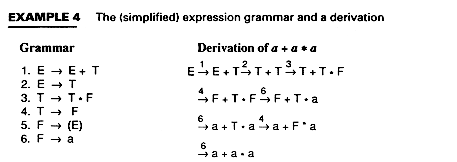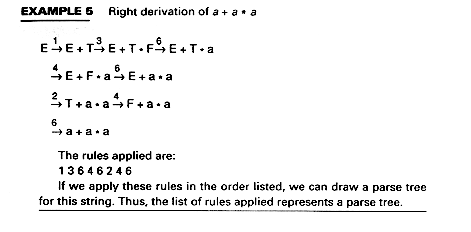# Derivations

The parse tree shows the structure, but it does not tell us in exactly what order the productions were applied. The following example shows the expression grammar and one derivation of a + a * a.

(E stands for expression, T for term and F for factor. The number above each arrow is the number of the production applied.

A left derivation replaces the left-most nonterminal at each step of the derivation, while a right derivation replaces the right-most nonterminal at each stepParsing reverses the derivation process: given an input string, the parser has to "discover" a derivation (if any).Send questions and comments to: Karen Lemone